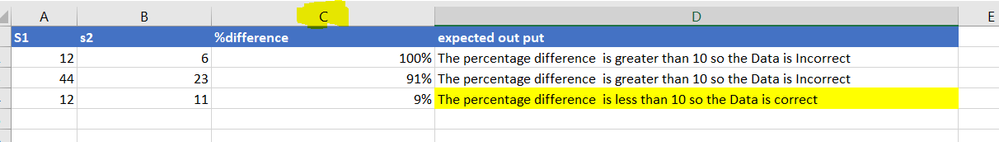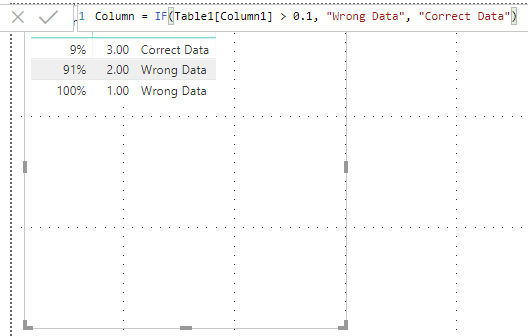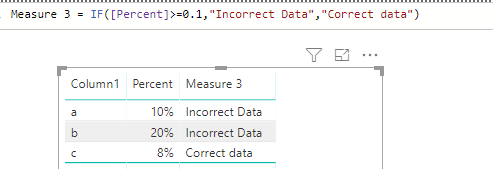cancel
Showing results for
Did you mean:Helper I

## How to create a new column on based on Percentage column

I have a table with a column that shows percentages as shown in the below image (column C), now I want to create a column on based on the percentage values in column C. For example, if the percentage is greater than or equal to 10 then output should "Incorrect Data" else if the percentage is less than 10 then output should be "correct data". How to achieve this condition column in Power BI on based on percentage values column?Thanks!

1 ACCEPTED SOLUTIONAnonymous
Not applicable
Relate to the values as decimals.
0.01 = 1%
0.1 = 10%
1.0 = 100%

Column = IF(Table1[Column1] > 0.1, "Wrong Data", "Correct Data")4 REPLIES 4Anonymous
Not applicable
Relate to the values as decimals.
0.01 = 1%
0.1 = 10%
1.0 = 100%

Column = IF(Table1[Column1] > 0.1, "Wrong Data", "Correct Data")Helper I

The percentage(%) column is created measure.Microsoft

Hi @ta5656

You may create a measure as below:

`Measure 3 = IF([Percent]>=0.1,"Incorrect Data","Correct data")`Regards,

Cherie

Community Support Team _ Cherie Chen
If this post helps, then please consider Accept it as the solution to help the other members find it more quickly.Helper I

@Anonymous Thanks for the response.

Can you guide me on how to relate values as decimals?

Thanks!